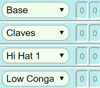# Binary DigitsA Binary Digit can only be 0 or 1

## Binary Number

A Binary Number is made up Binary Digits.In the computer world "binary digit" is often shortened to the word "bit"

## More Than One Digit

So, there are only two ways we can have a binary digit ("0" and "1", or "On" and "Off") ... but what about 2 or more binary digits?

Let's write them all down, starting with 1 digit (you can test it yourself using the switches):

2 ways to have one digit ...0 1
... 4 ways to have two digits ...0 0 → 00 1 → 01 1 0 → 10 1 → 11
... 8 ways to have three digits ...0 0 0 → 000 1 → 001 1 0 → 010 1 → 011 1 0 0 → 100 1 → 101 1 0 → 110 1 → 111
... and 16 ways to have four digits.0 0 0 0 → 0000 1 → 0001 1 0 → 0010 1 → 0011 1 0 0 → 0100 1 → 0101 1 0 → 0110 1 → 0111 1 0 0 0 → 1000 1 → 1001 1 0 → 1010 1 → 1011 1 0 0 → 1100 1 → 1101 1 0 → 1110 1 → 1111

Here is that last list sideways:

 0000 0001 0010 0011 0100 0101 0110 0111 1000 1001 1010 1011 1100 1101 1110 1111

And (without the leading 0s) we have the first 16 binary numbers:

 Binary: Decimal: 0 1 10 11 100 101 110 111 1000 1001 1010 1011 1100 1101 1110 1111 0 1 2 3 4 5 6 7 8 9 10 11 12 13 14 15

This is useful! To remember the sequence of binary numbers just think:

• "0" and "1" {0,1}
• then repeat "0" and "1" again but with a "1" in front: {0,1,10,11}
• then repeat those with a "1" in front: {0,1,10,11,100,101,110,111}
• and so on!

At each stage we repeat everything we have so far, but with a 1 in front.

Now find out how to use Binary to count past 1,000 on your fingers:

Activity: Binary FingersAlso have a Play with different drums.

## Binary Digits ... They Double!

Also notice that each time we add another binary digit we double the possible values.

Why double? Because we take all the previous possible values and match them with a "0" and a "1" like above.

• So just one binary digit has 2 possible values (0 and 1)
• Two binary digits have 4 possible values (0, 1, 10, 11)
• Three have 8 possible values
• Four have 16 possible values
• Five have 32 possible values
• Six have 64 possible values
• etc.

Using exponents, this can be shown as:

Number
of Digits
Formula Settings
1 21 2
2 22 4
3 23 8
4 24 16
5 25 32
6 26 64
etc... etc... etc...

### Example: when we have 50 binary digits (or 50 things that can only have two positions each), how many different ways is that?

Answer: 250 = 2 × 2 × 2 × 2 × 2 ... (fifty of these)
= 1,125,899,906,842,624

So, a binary number with 50 digits could have 1,125,899,906,842,624 different values.

Or to put it another way, it could show a number up to 1,125,899,906,842,623 (note: this is one less than the total number of values, because one of the values is 0).

### Example: Start the month with \$1 and double it every day, after 30 days you will be a billionaire!

230 = 2 × 2 × 2 × 2 ... (thirty of these)
= 1,073,741,824

## Chess BoardThere is an old Indian legend about a King who was challenged to a game of chess by a visiting Sage. The King asked "what is the prize if you win?".

The Sage said he would simply like some grains of rice: one on the first square, 2 on the second, 4 on the third and so on, doubling on each square. The King was surprised by this humble request.

Well, the Sage won, so how many grains of rice should he receive?

On the first square: 1 grain, on the second square: 2 grains (for a total of 3) and so on like this:

Square Grains Total
1 1 1
2 2 3
3 4 7
4 8 15
10 512 1,027
20 524,288
1,048,575
30 53,6870,912
1,073,741,823
64 ???
???

By the 30th square you can see it is already a lot of rice! A billion grains of rice is about 25 tonnes (1,000 grains is about 25g ... I weighed some!)

Notice that the Total of any square is 1 less than the Grains on the next square (Example: square 3's total is 7, and square 4 has 8 grains). So the total of all squares is a formula: 2n−1, where n is the number of the square. For example, for square 3, the total is 23−1 = 81 = 7

So, to fill all 64 squares in a chess board would need:

264−1 = 18,446,744,073,709,551,615 grains (460 billion tonnes of rice),

many times more rice than in the whole kingdom.

So, the power of binary doubling is nothing to be taken lightly (460 billion tonnes is not light!)Grains of rice on each square using scientific notation
Values are rounded off, so 53,6870,912 is shown as just 5×108
which means a 5 followed by 8 zeros

(By the way, in the legend the Sage reveals himself to be Lord Krishna and tells the King that he doesn't have to pay the debt at once, but can pay him over time, just serve rice to pilgrims every day until the debt is paid off.)